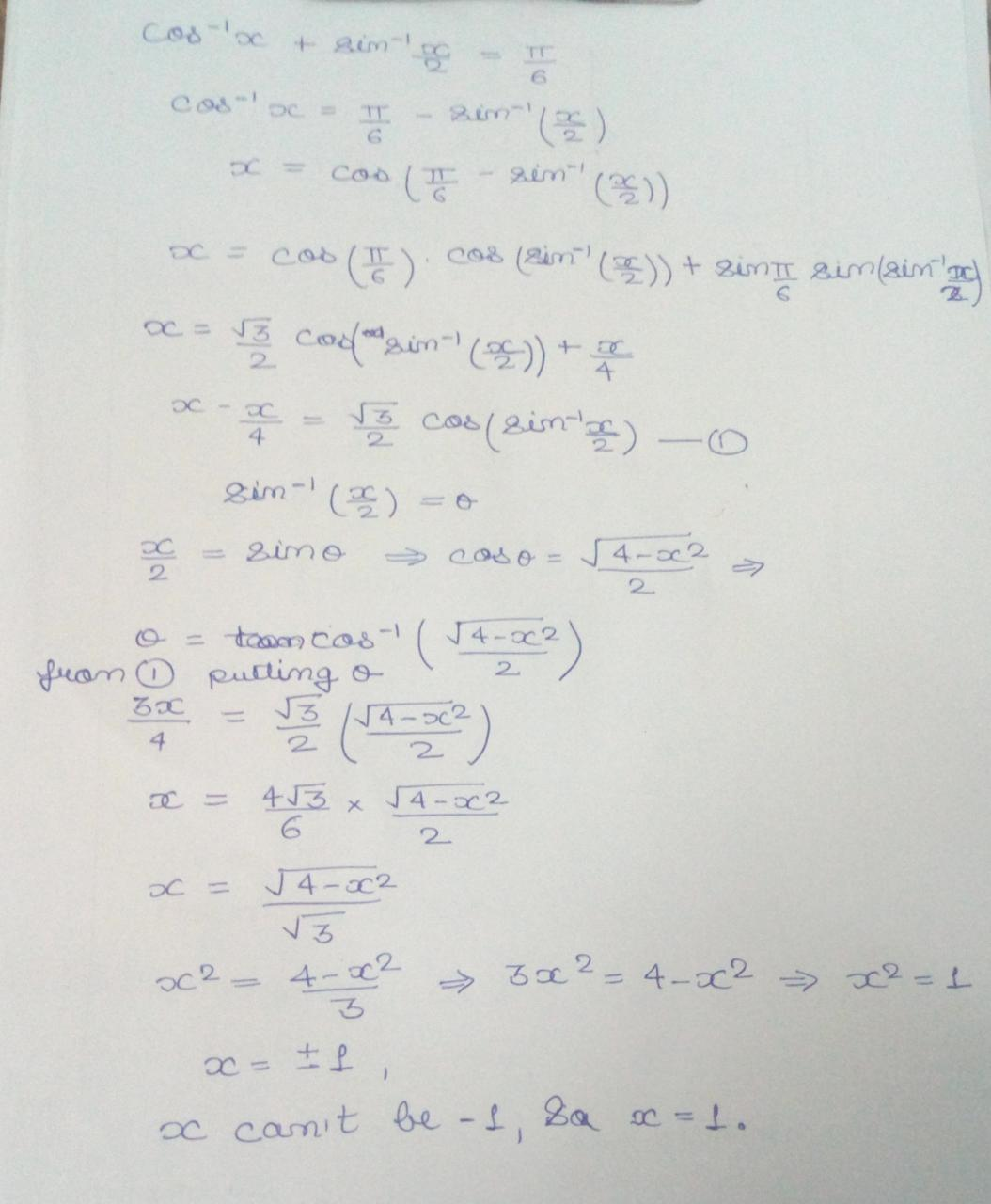×#### Thank you for registering.

One of our academic counsellors will contact you within 1 working day.

Click to Chat

1800-1023-196

+91-120-4616500

CART 0

• 0

MY CART (5)

Use Coupon: CART20 and get 20% off on all online Study Material

ITEM
DETAILS
MRP
DISCOUNT
FINAL PRICE
Total Price: Rs.

There are no items in this cart.
Continue Shopping
```
solve for x cos^-1(x) + sin^-1(x/2) = pi/6

```
6 years agoIIT Delhi
174 Points
```							take sin for both sides,sin(cos^-1(x) + sin^-1(x/2) )= sin(pi/6)sin(cos^-1(x))cos(sin^-1(x/2))+sin(sin^-1(x/2) ))cos(cos^-1(x))=.5sqrt(1-cos(cos^-1(x)))^2)sqrt(1-sin(sin^-1(x/2)))^2)+x^2/2=.5sqrt(1-x^2)sqrt(1-(x/2)^2)+x^2/2=.5now , solve for xsher mohammadb.tech, iit delhi
```
6 years ago
```							 We have cos–1x + sin–1 2x=6cos–1x =6– sin–1x x = cos  2x– sin6–1= cos6cos 2xsin–1+ sin6sin 2xsin–1 x =23cos 2x·214xcos 1–2–1    x =4x4x1–23 2 x –4x=4x1–23 24x1–2343x 2   4x1–43169x2 243x2= 1 –4x24x43x2 2 = 144x2= 1 x2 = 1  x = ±1 But x = 1
```
one year ago
```							Dear Student,Please find below the solution to your problem.Thanks and Regards
```
3 months ago
Think You Can Provide A Better Answer ?

## Other Related Questions on Trigonometry

View all Questions »### Course Features

• 731 Video Lectures
• Revision Notes
• Previous Year Papers
• Mind Map
• Study Planner
• NCERT Solutions
• Discussion Forum
• Test paper with Video Solution### Course Features

• 31 Video Lectures
• Revision Notes
• Test paper with Video Solution
• Mind Map
• Study Planner
• NCERT Solutions
• Discussion Forum
• Previous Year Exam Questions